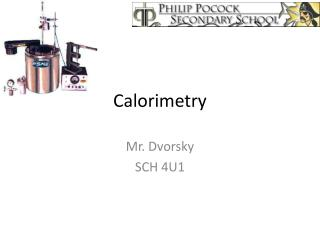DownloadDownload PresentationCalorimetry

# Calorimetry

Download Presentation## Calorimetry

- - - - - - - - - - - - - - - - - - - - - - - - - - - E N D - - - - - - - - - - - - - - - - - - - - - - - - - - -
##### Presentation Transcript

1. Calorimetry Mr. Dvorsky SCH 4U1

2. A calorimeter is an object used for measuring the heat of a chemical reaction or physical change. • Can be as simple as a pair of cups and a thermometer, or as complex as a device attached to the Large Hadron Collider.

3. Ideally, the calorimeter does not allow system to exchange either matter or energy with the surroundings (isolated). • There is water inside the calorimeter, and also a reaction or process of interest.

4. You can view it as water being one system and the process occurring in another system. They are in thermal contact but isolated from the rest of the universe.

5. Recall: The Laws of Thermodynamics • 1st Law – Energy of the Universe is constant so any energy added to the system came from the surroundings, and any energy lost by the system goes to the surroundings. • 2nd Law – Heat will pass from an object at higher temperature to cooler temperature until they reach thermal equilibrium.

6. Tying this to Calorimetry • A chemical reaction occurs in the calorimeter. • This results in a change in temp in the water system surrounding the reaction. • The enthalpy of reaction can be calculated based on the thermal energy transfer in the calorimeter.

7. Simple Calorimeter • -two stacked styrofoam • cups, lid & thermometer • -the reaction happens in • the inner cup with known • amount of water. • -the chemical reaction • usually occurs in the water

8. Key Assumptions • The system is isolated – no outside exchange of energy • The thermal energy exchanged to the cup and thermometer itself is so small it can be ignored. • If something reacts or dissolves in water in the calorimeter, the resulting solution still retains the properties of water (i.e. specific heat capacity). • Occurs at constant pressure

9. Once these assumptions are made… • The following applies: Thermal energy released by the system = -thermal energy absorbed by the surroundings Heat lost (or gained) by the system = -heat gained (or lost) by the surroundings. System = chemical change you are studying, and the surroundings = water in the calorimeter. Since the mass of water and its specific heat capacity is known, you can calculate the change in thermal energy using Q = mcΔT

10. Using calorimetry data to determine the enthalpy of reaction • Ideal for determingΔH of reactions in dilute aqueous solutions • The solution itself absorbs or releases the energy. ΔH = nΔHr

11. Flame and Bomb Calorimeters • Flame calorimeter can measure enthalpy of combustion of a substance that is burning. • Not all assumptions are the same, i.e. the heat absorbed by the calorimeter itself should be included in calculations • For pure substances, molar enthalpy of combustion is used. For foods, enthalpy of combustion is often expressed as kJ/g rather than kJ/mol. –see Figure 5.18 page 306

12. Bomb calorimeter – very precise, reaction takes place in a bomb. -see clip.